MORE IN Analog Electronics - 1
MU Electronics and Telecom Engineering (Semester 3)
Analog Electronics - 1
May 2014
Total marks: --
Total time: --
INSTRUCTIONS
(1) Assume appropriate data and state your reasons
(2) Marks are given to the right of every question
(3) Draw neat diagrams wherever necessary

Solve any five :-
1 (a) Explain effect of temperature on characteristics of PN junction diode.
4 M
1 (b) Why LC oscillators are prefered for high frequency applications?
4 M
1 (c) Find RB and RC for the circuit shown to obtain VCE=5V and IC=2mA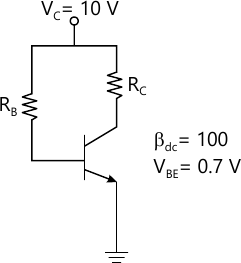4 M
1 (d) In n-channel MOSFET VDS=5V, VGS=5V, VBS=0, W=10 ?m, L=5 ?m k'n=100 mA/V2 and VTO=1V. Calculate its drain current for channel length modulation factor ? of 0 and 0.25 V-1
4 M
1 (e) Draw and explain small signal hybrid-Pi model of BJT including early effect.
4 M
1 (f) Differentiate between NJT and MOSFET
4 M

2 (a) Find ICQ and VCEQ for the circuit shown in figure 2a if ?=100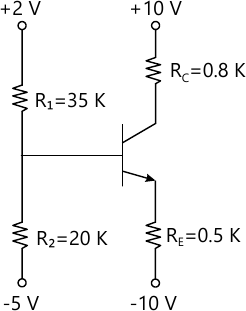10 M
2 (b) Draw and explain energy band diagram of MOS capacitor in accumulation, depletion and inversion region.
10 M

3 (a) Draw and explain working of transisterized Wien Bridge Oscillator
10 M
3 (b) The JFET shown in figure 3b has parameter IDSS=8mA and Vp=4V. Determine VG, IDSQ, VGSQ and VDSQ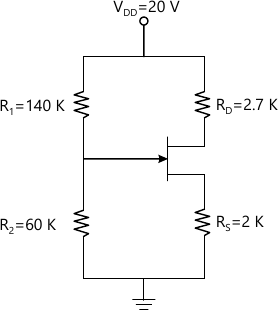10 M

4 (a) For the common gate circuit shown in figure 4s, the NMOS transistor parameters are VTN=1, kn=3 mA/V2 and ?=0 (i) Determine IDSQ and VDSQ (ii) Calculate gm and ro (iii) Find the small signal voltage gain Av= v0/v1. Assume Cc1 and Cc2 acts as short circuit for small-signal analysis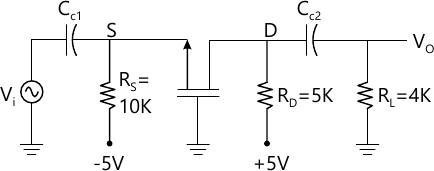10 M
4 (b) The parameter of the transistor in the circuit shown in figure 4b are ?=100 and VA=100V. (i) Determine the voltages at base and emitter terminals (ii) Find Rc such that VCEQ=3.5V and (iii) Assuming CC and CE acts as short circuit determine small signal voltages gain Av= v0/vs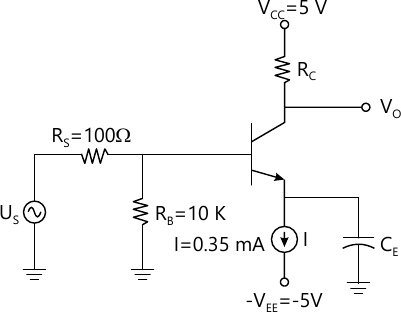10 M

5 (a) Derive expression for voltage gain NMOS source follower cicuit
8 M
5 (b) For the common base amplifier shown in figure 5b, derive expression for voltage gain, current gain, input resistance and output resistance using hybrid-? model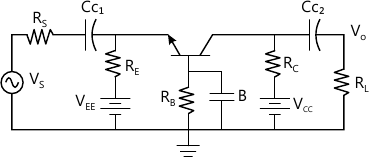12 M

Write short note on any three :-
6 (a) Series and shunt clippers
7 M
6 (b) Twin-T oscillator
7 M
6 (c) MOSFET operation
7 M
6 (d) Construction and operation of varactor diode.
7 M

More question papers from Analog Electronics - 1